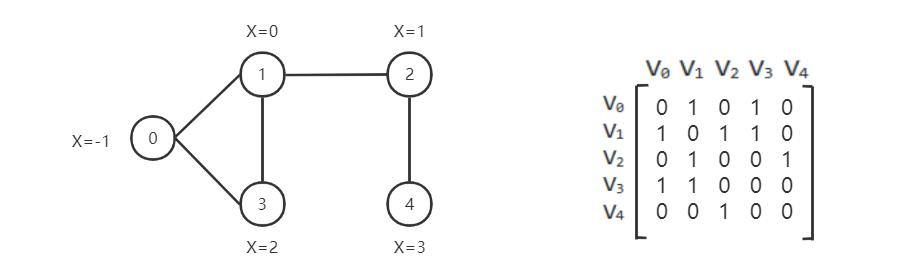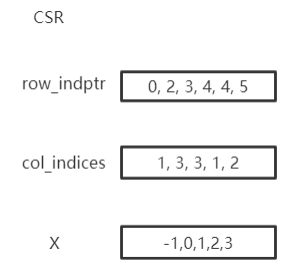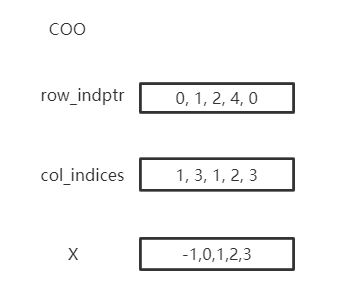# Introduction to Graphs

## Real-world graphs

Graph-structured data have been widely utilized in many real-world scenarios. For example, each user on Facebook can be seen as a vertex and their relations like friendship or followership can be seen as edges in the graph. We might be interested in predicting the interests of users, or whether a pair of nodes in a network might have an edge connecting them.

A graph can be represented using an adjacency matrix## How to represent a graph in CogDL

A graph is used to store information of structured data. CogDL represents a graph with a cogdl.data.Graph object. Briefly, a Graph holds the following attributes:

• x: Node feature matrix with shape [num_nodes, num_features], torch.Tensor

• edge_index: COO format sparse matrix, Tuple

• edge_weight: Edge weight with shape [num_edges,], torch.Tensor

• edge_attr: Edge attribute matrix with shape [num_edges, num_attr]

• y: Target labels of each node, with shape [num_nodes,] for single label case and [num_nodes, num_labels] for mult-label case

• row_indptr: Row index pointer for CSR sparse matrix, torch.Tensor.

• col_indices: Column indices for CSR sparse matrix, torch.Tensor.

• num_nodes: The number of nodes in graph.

• num_edges: The number of edges in graph.

The above are the basic attributes but are not necessary. You may define a graph with g = Graph(edge_index=edges) and omit the others. Besides, Graph is not restricted to these attributes and other self-defined attributes, e.g., graph.mask = mask, are also supported.

Represent this graph in cogdl:Graph stores sparse matrix with COO or CSR format. COO format is easier to add or remove edges, e.x. add_self_loops, and CSR is stored for fast message-passing. Graph automatically convert between two formats and you can use both on demands without worrying. You can create a Graph with edges or assign edges to a created graph. edge_weight will be automatically initialized as all ones, and you can modify it to fit your need.

import torch
from cogdl.data import Graph
edges = torch.tensor([[0,1],[1,3],[2,1],[4,2],[0,3]]).t()
x = torch.tensor([[-1],,,,])
g = Graph(edge_index=edges,x=x) # equivalent to that above
print(g.row_indptr)
>>tensor([0, 2, 3, 4, 4, 5])
print(g.col_indices)
>>tensor([1, 3, 3, 1, 2])
print(g.edge_weight)
>> tensor([1., 1., 1., 1., 1.])
g.num_nodes
>> 5
g.num_edges
>> 5
g.edge_weight = torch.rand(5)
print(g.edge_weight)
>> tensor([0.8399, 0.6341, 0.3028, 0.0602, 0.7190])


We also implement commonly used operations in Graph:

• add_self_loops: add self loops for nodes in graph,

$\hat{A}=A+I$
• add_remaining_self_loops: add self-loops for nodes without it.

• sym_norm: symmetric normalization of edge_weight used GCN:

$\hat{A}=D^{-1/2}AD^{-1/2}$
• row_norm: row-wise normalization of edge_weight:

$\hat{A} = D^{-1}A$
• degrees: get degrees for each node. For directed graph, this function returns in-degrees of each node.

import torch
from cogdl.data import Graph
edge_index = torch.tensor([[0,1],[1,3],[2,1],[4,2],[0,3]]).t()
g = Graph(edge_index=edge_index)
>> Graph(edge_index=[2, 5])
>> Graph(edge_index=[2, 10], edge_weight=)
>> print(edge_weight) # tensor([1., 1., ..., 1.])
g.row_norm()
>> print(edge_weight) # tensor([0.3333, ..., 0.50])

• subgraph: get a subgraph containing given nodes and edges between them.

• edge_subgraph: get a subgraph containing given edges and corresponding nodes.

• sample_adj: sample a fixed number of neighbors for each given node.

from cogdl.datasets import build_dataset_from_name
g = build_dataset_from_name("cora")
g.num_nodes
>> 2708
g.num_edges
>> 10556
# Get a subgraph contaning nodes [0, .., 99]
sub_g = g.subgraph(torch.arange(100))
>> Graph(x=[100, 1433], edge_index=[2, 18], y=)
# Sample 3 neighbors for each nodes in [0, .., 99]

• train/eval: In inductive settings, some nodes and edges are unseen during training, train/eval provides access to switching backend graph for training/evaluation. In transductive setting, you may ignore this.

# train_step
model.train()
graph.train()

# inference_step
model.eval()
graph.eval()


## How to construct mini-batch graphs

In node classification, all operations are in one single graph. But in tasks like graph classification, we need to deal with many graphs with mini-batch. Datasets for graph classification contains graphs which can be accessed with index, e.x. data. To support mini-batch training/inference, CogDL combines graphs in a batch into one whole graph, where adjacency matrices form sparse block diagnal matrices and others(node features, labels) are concatenated in node dimension. cogdl.data.Dataloader handles the process.

from cogdl.data import DataLoader
from cogdl.datasets import build_dataset_from_name

dataset = build_dataset_from_name("mutag")
>> MUTAGDataset(188)
dataset
>> Graph(x=[17, 7], y=, edge_index=[2, 38])
model(batch)
>> Batch(x=[154, 7], y=, batch=, edge_index=[2, 338])


batch is an additional attributes that indicate the respective graph the node belongs to. It is mainly used to do global pooling, or called readout to generate graph-level representation. Concretely, batch is a tensor like:

$batch=[0,..,0, 1,...,1, N-1,...,N-1]$

The following code snippet shows how to do global pooling to sum over features of nodes in each graph:

def batch_sum_pooling(x, batch):
batch_size = int(torch.max(batch.cpu())) + 1
res = torch.zeros(batch_size, x.size(1)).to(x.device)
dim=0,
index=batch.unsqueeze(-1).expand_as(x),
src=x
)
return out


## How to edit the graph?

Changes can be applied to edges in some settings. In such cases, we need to generate a graph for calculation while keep the original graph. CogDL provides graph.local_graph to set up a local scape and any out-of-place operation will not reflect to the original graph. However, in-place operation will affect the original graph.

graph = build_dataset_from_name("cora")
graph.num_edges
>> 10556
with graph.local_graph():
row, col = graph.edge_index
graph.num_edges
>> 100
graph.num_edges
>> 10556

graph.edge_weight
>> tensor([1.,...,1.])
with graph.local_graph():
graph.edge_weight += 1
graph.edge_weight
>> tensor([2.,...,2.])


## Common graph datasets

CogDL provides a bunch of commonly used datasets for graph tasks like node classification, graph classification and others. You can access them conveniently shown as follows.

from cogdl.datasets import build_dataset_from_name
dataset = build_dataset_from_name("cora")

from cogdl.datasets import build_dataset
dataset = build_dataset(args) # if args.dataet = "cora"


For all datasets for node classification, we use train_mask, val_mask, test_mask to denote train/validation/test split for nodes.

CogDL now supports the following datasets for different tasks:

• Network Embedding (Unsupervised node classification): PPI, Blogcatalog, Wikipedia, Youtube, DBLP, Flickr

• Semi/Un-superviesd Node classification: Cora, Citeseer, Pubmed, Reddit, PPI, PPI-large, Yelp, Flickr, Amazon

• Heterogeneous node classification: DBLP, ACM, IMDB

• Link prediction: PPI, Wikipedia, Blogcatalog

• graph classification: MUTAG, IMDB-B, IMDB-M, PROTEINS, COLLAB, NCI, NCI109, Reddit-BINARY

Dataset

Nodes

Edges

Classes

Degree

Name in Cogdl

PPI

3,890

76,584

50(m)

ppi-ne

BlogCatalog

10,312

333,983

40(m)

32

blogcatalog

Wikipedia

4.777

184,812

39(m)

39

wikipedia

Flickr

80,513

5,899,882

195(m)

73

flickr-ne

DBLP

51,264

2,990,443

60(m)

2

dblp-ne

1,138,499

2,990,443

47(m)

3

### Node classification

Dataset

Nodes

Edges

Features

Classes

Train/Val/Test

Degree

Name in cogdl

Cora

2,708

5,429

1,433

7(s)

140 / 500 / 1000

2

cora

Citeseer

3,327

4,732

3,703

6(s)

120 / 500 / 1000

1

citeseer

PubMed

19,717

44,338

500

3(s)

60 / 500 / 1999

2

pubmed

Chameleon

2,277

36,101

2,325

5

0.48 / 0.32 / 0.20

16

chameleon

Cornell

183

298

1,703

5

0.48 / 0.32 / 0.20

1.6

cornell

Film

7,600

30,019

932

5

0.48 / 0.32 / 0.20

4

film

Squirrel

5201

217,073

2,089

5

0.48 / 0.32 / 0.20

41.7

squirrel

Texas

182

325

1,703

5

0.48 / 0.32 / 0.20

1.8

texas

Wisconsin

251

515

1,703

5

0.48 / 0.32 / 0.20

2

Wisconsin

PPI

14,755

225,270

50

121(m)

0.66 / 0.12 / 0.22

15

ppi

PPI-large

56,944

818,736

50

121(m)

0.79 / 0.11 / 0.10

14

ppi-large

Reddit

232,965

11,606,919

602

41(s)

0.66 / 0.10 / 0.24

50

reddit

Flickr

89,250

899,756

500

7(s)

0.50 / 0.25 / 0.25

10

flickr

Yelp

716,847

6,977,410

300

100(m)

0.75 / 0.10 / 0.15

10

yelp

Amazon-SAINT

1,598,960

132,169,734

200

107(m)

0.85 / 0.05 / 0.10

83

amazon-s

### Heterogenous Graph

Dataset

Nodes

Edges

Features

Classes

Train/Val/Test

Degree

Edge Type

Name in Cogdl

DBLP

18,405

67,946

334

4

800 / 400 / 2857

4

4

gtn-dblp(han-acm)

ACM

8,994

25,922

1,902

3

600 / 300 / 2125

3

4

gtn-acm(han-acm)

IMDB

12,772

37,288

1,256

3

300 / 300 / 2339

3

4

gtn-imdb(han-imdb)

Amazon-GATNE

10,166

148,863

15

2

amazon

2,000

1,310,617

655

5

10,000

331,899

33

4

### Graph Classification

TUdataset from https://www.chrsmrrs.com/graphkerneldatasets

Dataset

Graphs

Classes

Avg. Size

Name in Cogdl

MUTAG

188

2

17.9

mutag

IMDB-B

1,000

2

19.8

imdb-b

IMDB-M

1,500

3

13

imdb-m

PROTEINS

1,113

2

39.1

proteins

COLLAB

5,000

5

508.5

collab

NCI1

4,110

2

29.8

nci1

NCI109

4,127

2

39.7

nci109

PTC-MR

344

2

14.3

ptc-mr

REDDIT-BINARY

2,000

2

429.7

reddit-b

REDDIT-MULTI-5k

4,999

5

508.5

reddit-multi-5k

REDDIT-MULTI-12k

11,929

11

391.5

reddit-multi-12k

BBBP

2,039

2

24

bbbp

BACE

1,513

2

34.1

bace